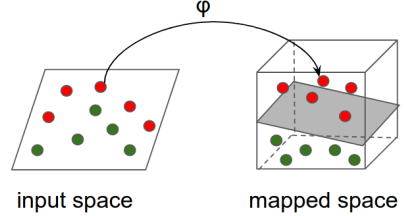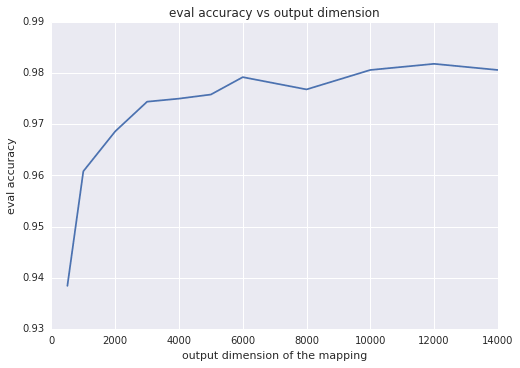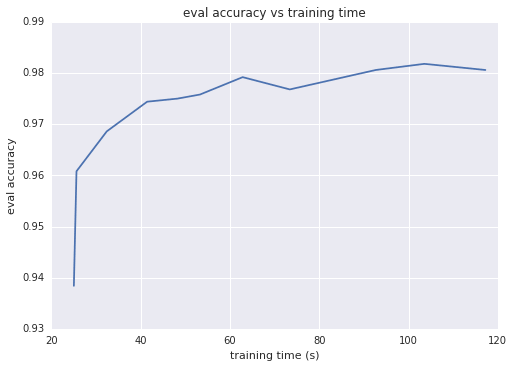# W3cubDocs

/TensorFlow Guide

# Improving Linear Models Using Explicit Kernel Methods

Note: This document uses a deprecated version of tf.estimator, which has a different interface. It also uses other contrib methods whose API may not be stable.

In this tutorial, we demonstrate how combining (explicit) kernel methods with linear models can drastically increase the latters' quality of predictions without significantly increasing training and inference times. Unlike dual kernel methods, explicit (primal) kernel methods scale well with the size of the training dataset both in terms of training/inference times and in terms of memory requirements.

Intended audience: Even though we provide a high-level overview of concepts related to explicit kernel methods, this tutorial primarily targets readers who already have at least basic knowledge of kernel methods and Support Vector Machines (SVMs). If you are new to kernel methods, refer to either of the following sources for an introduction:

Currently, TensorFlow supports explicit kernel mappings for dense features only; TensorFlow will provide support for sparse features at a later release.

This tutorial uses tf.contrib.learn (TensorFlow's high-level Machine Learning API) Estimators for our ML models. If you are not familiar with this API, tf.estimator Quickstart is a good place to start. We will use the MNIST dataset. The tutorial consists of the following steps:

• Load and prepare MNIST data for classification.
• Construct a simple linear model, train it, and evaluate it on the eval data.
• Replace the linear model with a kernelized linear model, re-train, and re-evaluate.

## Load and prepare MNIST data for classification

Run the following utility command to load the MNIST dataset:

data = tf.contrib.learn.datasets.mnist.load_mnist()


The preceding method loads the entire MNIST dataset (containing 70K samples) and splits it into train, validation, and test data with 55K, 5K, and 10K samples respectively. Each split contains one numpy array for images (with shape [sample_size, 784]) and one for labels (with shape [sample_size, 1]). In this tutorial, we only use the train and validation splits to train and evaluate our models respectively.

In order to feed data to a tf.contrib.learn Estimator, it is helpful to convert it to Tensors. For this, we will use an input function which adds Ops to the TensorFlow graph that, when executed, create mini-batches of Tensors to be used downstream. For more background on input functions, check this section on input functions. In this example, we will use the tf.train.shuffle_batch Op which, besides converting numpy arrays to Tensors, allows us to specify the batch_size and whether to randomize the input every time the input_fn Ops are executed (randomization typically expedites convergence during training). The full code for loading and preparing the data is shown in the snippet below. In this example, we use mini-batches of size 256 for training and the entire sample (5K entries) for evaluation. Feel free to experiment with different batch sizes.

import numpy as np
import tensorflow as tf

def get_input_fn(dataset_split, batch_size, capacity=10000, min_after_dequeue=3000):

def _input_fn():
images_batch, labels_batch = tf.train.shuffle_batch(
tensors=[dataset_split.images, dataset_split.labels.astype(np.int32)],
batch_size=batch_size,
capacity=capacity,
min_after_dequeue=min_after_dequeue,
enqueue_many=True,
features_map = {'images': images_batch}
return features_map, labels_batch

return _input_fn

train_input_fn = get_input_fn(data.train, batch_size=256)
eval_input_fn = get_input_fn(data.validation, batch_size=5000)



## Training a simple linear model

We can now train a linear model over the MNIST dataset. We will use the tf.contrib.learn.LinearClassifier estimator with 10 classes representing the 10 digits. The input features form a 784-dimensional dense vector which can be specified as follows:

image_column = tf.contrib.layers.real_valued_column('images', dimension=784)


The full code for constructing, training and evaluating a LinearClassifier estimator is as follows:

import time

# Specify the feature(s) to be used by the estimator.
image_column = tf.contrib.layers.real_valued_column('images', dimension=784)
estimator = tf.contrib.learn.LinearClassifier(feature_columns=[image_column], n_classes=10)

# Train.
start = time.time()
estimator.fit(input_fn=train_input_fn, steps=2000)
end = time.time()
print('Elapsed time: {} seconds'.format(end - start))

# Evaluate and report metrics.
eval_metrics = estimator.evaluate(input_fn=eval_input_fn, steps=1)
print(eval_metrics)


The following table summarizes the results on the eval data.

metric value
loss 0.25 to 0.30
accuracy 92.5%
training time ~25 seconds on my machine
Note: Metrics will vary depending on various factors.

In addition to experimenting with the (training) batch size and the number of training steps, there are a couple other parameters that can be tuned as well. For instance, you can change the optimization method used to minimize the loss by explicitly selecting another optimizer from the collection of available optimizers. As an example, the following code constructs a LinearClassifier estimator that uses the Follow-The-Regularized-Leader (FTRL) optimization strategy with a specific learning rate and L2-regularization.

optimizer = tf.train.FtrlOptimizer(learning_rate=5.0, l2_regularization_strength=1.0)
estimator = tf.contrib.learn.LinearClassifier(
feature_columns=[image_column], n_classes=10, optimizer=optimizer)


Regardless of the values of the parameters, the maximum accuracy a linear model can achieve on this dataset caps at around 93%.

## Using explicit kernel mappings with the linear model.

The relatively high error (~7%) of the linear model over MNIST indicates that the input data is not linearly separable. We will use explicit kernel mappings to reduce the classification error.

Intuition: The high-level idea is to use a non-linear map to transform the input space to another feature space (of possibly higher dimension) where the (transformed) features are (almost) linearly separable and then apply a linear model on the mapped features. This is shown in the following figure:### Technical details

In this example we will use Random Fourier Features, introduced in the "Random Features for Large-Scale Kernel Machines" paper by Rahimi and Recht, to map the input data. Random Fourier Features map a vector $$\mathbf{x} \in \mathbb{R}^d$$ to $$\mathbf{x'} \in \mathbb{R}^D$$ via the following mapping:

$$RFFM(\cdot): \mathbb{R}^d \to \mathbb{R}^D, \quad RFFM(\mathbf{x}) = \cos(\mathbf{\Omega} \cdot \mathbf{x}+ \mathbf{b})$$

where $$\mathbf{\Omega} \in \mathbb{R}^{D \times d}$$, $$\mathbf{x} \in \mathbb{R}^d,$$ $$\mathbf{b} \in \mathbb{R}^D$$ and the cosine is applied element-wise.

In this example, the entries of $$\mathbf{\Omega}$$ and $$\mathbf{b}$$ are sampled from distributions such that the mapping satisfies the following property:

$$RFFM(\mathbf{x})^T \cdot RFFM(\mathbf{y}) \approx e^{-\frac{\|\mathbf{x} - \mathbf{y}\|^2}{2 \sigma^2}}$$

The right-hand-side quantity of the expression above is known as the RBF (or Gaussian) kernel function. This function is one of the most-widely used kernel functions in Machine Learning and implicitly measures similarity in a different, much higher dimensional space than the original one. See Radial basis function kernel for more details.

### Kernel classifier

tf.contrib.kernel_methods.KernelLinearClassifier is a pre-packaged tf.contrib.learn estimator that combines the power of explicit kernel mappings with linear models. Its constructor is almost identical to that of the LinearClassifier estimator with the additional option to specify a list of explicit kernel mappings to be applied to each feature the classifier uses. The following code snippet demonstrates how to replace LinearClassifier with KernelLinearClassifier.

# Specify the feature(s) to be used by the estimator. This is identical to the
# code used for the LinearClassifier.
image_column = tf.contrib.layers.real_valued_column('images', dimension=784)
optimizer = tf.train.FtrlOptimizer(
learning_rate=50.0, l2_regularization_strength=0.001)

kernel_mapper = tf.contrib.kernel_methods.RandomFourierFeatureMapper(
input_dim=784, output_dim=2000, stddev=5.0, name='rffm')
kernel_mappers = {image_column: [kernel_mapper]}
estimator = tf.contrib.kernel_methods.KernelLinearClassifier(
n_classes=10, optimizer=optimizer, kernel_mappers=kernel_mappers)

# Train.
start = time.time()
estimator.fit(input_fn=train_input_fn, steps=2000)
end = time.time()
print('Elapsed time: {} seconds'.format(end - start))

# Evaluate and report metrics.
eval_metrics = estimator.evaluate(input_fn=eval_input_fn, steps=1)
print(eval_metrics)


The only additional parameter passed to KernelLinearClassifier is a dictionary from feature_columns to a list of kernel mappings to be applied to the corresponding feature column. The following lines instruct the classifier to first map the initial 784-dimensional images to 2000-dimensional vectors using random Fourier features and then learn a linear model on the transformed vectors:

kernel_mapper = tf.contrib.kernel_methods.RandomFourierFeatureMapper(
input_dim=784, output_dim=2000, stddev=5.0, name='rffm')
kernel_mappers = {image_column: [kernel_mapper]}
estimator = tf.contrib.kernel_methods.KernelLinearClassifier(
n_classes=10, optimizer=optimizer, kernel_mappers=kernel_mappers)


Notice the stddev parameter. This is the standard deviation ($$\sigma$$) of the approximated RBF kernel and controls the similarity measure used in classification. stddev is typically determined via hyperparameter tuning.

The results of running the preceding code are summarized in the following table. We can further increase the accuracy by increasing the output dimension of the mapping and tuning the standard deviation.

metric value
loss 0.10
accuracy 97%
training time ~35 seconds on my machine

### stddev

The classification quality is very sensitive to the value of stddev. The following table shows the accuracy of the classifier on the eval data for different values of stddev. The optimal value is stddev=5.0. Notice how too small or too high stddev values can dramatically decrease the accuracy of the classification.

stddev eval accuracy
1.0 0.1362
2.0 0.4764
4.0 0.9654
5.0 0.9766
8.0 0.9714
16.0 0.8878

### Output dimension

Intuitively, the larger the output dimension of the mapping, the closer the inner product of two mapped vectors approximates the kernel, which typically translates to better classification accuracy. Another way to think about this is that the output dimension equals the number of weights of the linear model; the larger this dimension, the larger the "degrees of freedom" of the model. However, after a certain threshold, higher output dimensions increase the accuracy by very little, while making training take more time. This is shown in the following two Figures which depict the eval accuracy as a function of the output dimension and the training time, respectively.## Summary

Explicit kernel mappings combine the predictive power of nonlinear models with the scalability of linear models. Unlike traditional dual kernel methods, explicit kernel methods can scale to millions or hundreds of millions of samples. When using explicit kernel mappings, consider the following tips:

• Random Fourier Features can be particularly effective for datasets with dense features.
• The parameters of the kernel mapping are often data-dependent. Model quality can be very sensitive to these parameters. Use hyperparameter tuning to find the optimal values.
• If you have multiple numerical features, concatenate them into a single multi-dimensional feature and apply the kernel mapping to the concatenated vector.